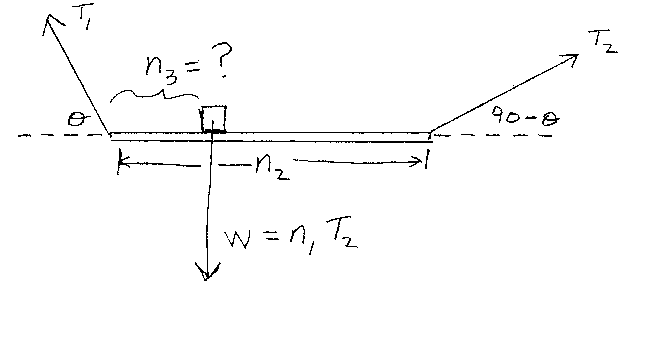Problem A14: In the figure below, a weightless plank has a weight W resting on it. It is held up by two strings having tensions of T1 and T2. The left string makes an angle &theta degrees with the horizontal, and the right string makes an angle of (90-&theta) degrees with the horizontal. The weight W has a value of W = n1 T2. The plank is n2 units long. What is the distance n3 that the weight W is from the left end of the plank? n3 = ? Note that n1 is unitless, and that n2 and n3 have the same units of length.n1 = n2 = Input n3:
If you are currently in my class, you can record your grade by entering your name and student ID number (without the leading zeros) below and clicking on "record grade".
 First Name = Last Name = ID = Problem: